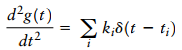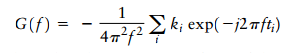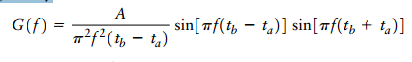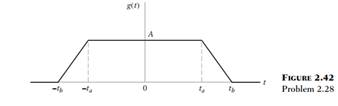Create an Account

Home / Questions / Consider a pulselike function that consists of a small number of straight line segments Su...

Consider a pulselike function that consists of a small number of straight line segments Suppose that this function is differentiated with respect to time t twice so as to generate a sequence of

Consider a pulselike function that consists of a small number of straight-line segments. Suppose that this function is differentiated with respect to time t twice so as to generate a sequence of weighted delta functions, as shown bywhere the are related to the slopes of the straight-line segments.

(a) Given the values of the and show that the Fourier transform of is given by(b) Using this procedure, show that the Fourier transform of the trapezoidal pulse shown in Fig. 2.42 is given byJul 31 2020 View more View LessSubscribe To Get Solution